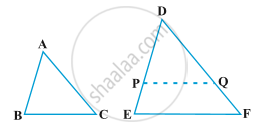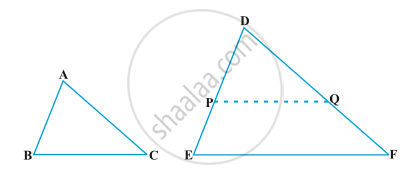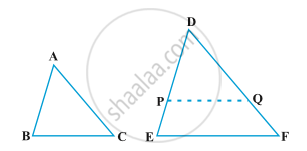Karnataka SSLC Karnataka Secondary Education Examination Board
Share

# Criteria for Similarity of Triangles

#### notes

Already in concept 2 we have learned the criteria for similarityof triangles, let's recall it.
Two figures are similar if
1) The corresponding angles are same in measurement i.e ∠A≅∠D, ∠B≅∠E, ∠C≅∠F AND

2) The corresponding sides are of same ratios i.e "AB"/"DE"="BC"/"EF"= "AC"/"DF"
In this concept we will learn three new theorems, let's understand them one by one.

#### theorem

Theorem1:  If in two triangles, corresponding angles are equal, then their corresponding sides are in the same ratio (or proportion) and hence the two triangles are similar.Given: ∠A= ∠D, ∠B= ∠E, ∠C= ∠F

To prove: "AB"/"DE"="BC"/"EF"= "AC"/"DF"

Construction: Cut DP=AB, DQ=AC, join PQ
Proof: This criterion is referred to as the AAA (Angle–Angle–Angle) criterion of similarity of two triangles.
In ∆ABC and ∆DPQ
AB= DP (by construction)
AC= DQ (by construction)
∠A= ∠D (Given)
∆ABC ≅ ∆DPQ (Side-Angle-Side rule)
Side-Angle-Side is a rule used to prove whether a given set of triangles are congruent. If two sides and the included angle of one triangle are equal to two sides and included angle of another triangle, then the triangles are congruent. An included angle is an angle formed by two given sides.
So, ∠B= ∠P (Corresponding parts of Congruent triangles)
But, ∠B= ∠E (Given)
Therefore, ∠P= ∠E, ∠P and ∠E are corresponding to each other.
Now, we know that if corresponding are equal then the lines are parallel.
As per this rule, if ∠P= ∠E,
then PQ is parallel to EF

"DP"/"PE" =" DQ"/"QF" (by BPT)

We can also say that, "DP"/"DE"= "DQ"/"DF" (by corollary of BPT) AB=DP (by construction)

Therefore, "AB"/"DE"= "AC"/"DF" ........eq1

Similarly, if draw a line MN and prove it parallel to DF

Then "BC"/"EF"= "AB"/"DE" ..........eq2

And also "AC"/"DF"= "BC"/"EF" ..........eq3

From eq1, eq2 and eq3

"AB"/"DE" = "BC"/"EF" = "AC"/"DF"

hence, the two triangles are similar.

Theorem2:  If in two triangles, sides of one triangle are proportional to (i.e., in the same ratio of) the sides of the other triangle, then their corresponding angles are equal and hence the two triangles are similiar.
This criterion is referred to as the SSS (Side–Side–Side) similarity criterion for two triangles.Given: "AB"/"DE"= "BC"/"EF"= "AC"/"DF"

To prove: ∠A= ∠D, ∠B= ∠E, ∠C= ∠F
Construction: Cut DP=AB and DQ=AC, join PQ

Proof: "AB"/"DE"= "AC"/"DF" (Given)

"DP"/"DE"= "DQ"/"DF" (by construction)

PQ || EF (converse of BPT)
∠P= ∠E (corresponding angles)
∠Q= ∠F (corresponding angles)
Now two corresponding angles of two triangles are equal, ∆DPQ ∼ ∆DEF by AA Similarity test.

Therefore, "DP"/"DE" = "PQ"/"EF" (Corresponding parts of Congruent triangles)

"AB"/"DE"= "PQ"/"EF" [Since, AB=DP]

"BC"/"EF"= "PQ"/"EF" ("AB"/"DE"= "BC"/"EF"  "is"  "given")

"BC" = "PQ"
In ∆ABC and ∆DPQ,
DP=AB  (by construction)
DQ=AC  (by construction)
BC= PQ (proved above)
Therefore, ∆ABC ≅ ∆DPQ (Side-Side-Side rule)
∠A= ∠D (Corresponding parts of Congruent triangles)
∠B= ∠P (Corresponding parts of Congruent triangles)
∠C= ∠Q (Corresponding parts of Congruent triangles)
But ∠P= ∠E
Therefore, ∠B= ∠E
And ∠Q= ∠F
Therefore, ∠C= ∠E
Hence, the two triangles are similar.

Theorem3: If one angle of a triangle is equal to one angle of the other triangle and the sides including these angles are proportional, then the two triangles are similar.
This criterion is referred to as the SAS (Side–Angle–Side) similarity criterion for two triangles.Given: ∠A= ∠D, "AB"/"DE" ="AC"/"DF"

To prove: ∆ABC ∼ ∆DEF
Construction: Let us take the ∆ABC and ∆DEF such that

"AB"/"DE" = "AC"/"DF" = <1

Cut DP=AB and DQ=AC, join PQ.
Proof: In ∆ABC and ∆DPQ,
AB= DP (by construction)
∠A= ∠D (given)
AC= DQ (by construction)
Therefore, ∆ABC ≅ ∆DPQ (Side-Angle-Side rule)
∠B= ∠P (Corresponding parts of Congruent triangles)
∠C= ∠Q (Corresponding parts of Congruent triangles)

"AB"/"DE" = "AC"/"DF" (Given)

"DP"/"DE" = "DQ"/"DF"  [Since, DP=AB and DQ=AC]

PQ || EF (by converse of BPT)
∠P= ∠E and ∠Q= ∠F (corresponding angles)
∠P= ∠B and ∠Q= ∠C (proved above)
Therefore, ∠B= ∠E and ∠C= ∠F
Therefore, the two triangles are equiangular.
So, ∆ABC ∼ ∆DEF
Hence proved.

#### Video Tutorials

We have provided more than 1 series of video tutorials for some topics to help you get a better understanding of the topic.

Series 1

Series 2

Series 3

### Shaalaa.com

Triangles part 18 (AAA Angle Angle Angle Similarity) [00:12:35]
S
0%

S